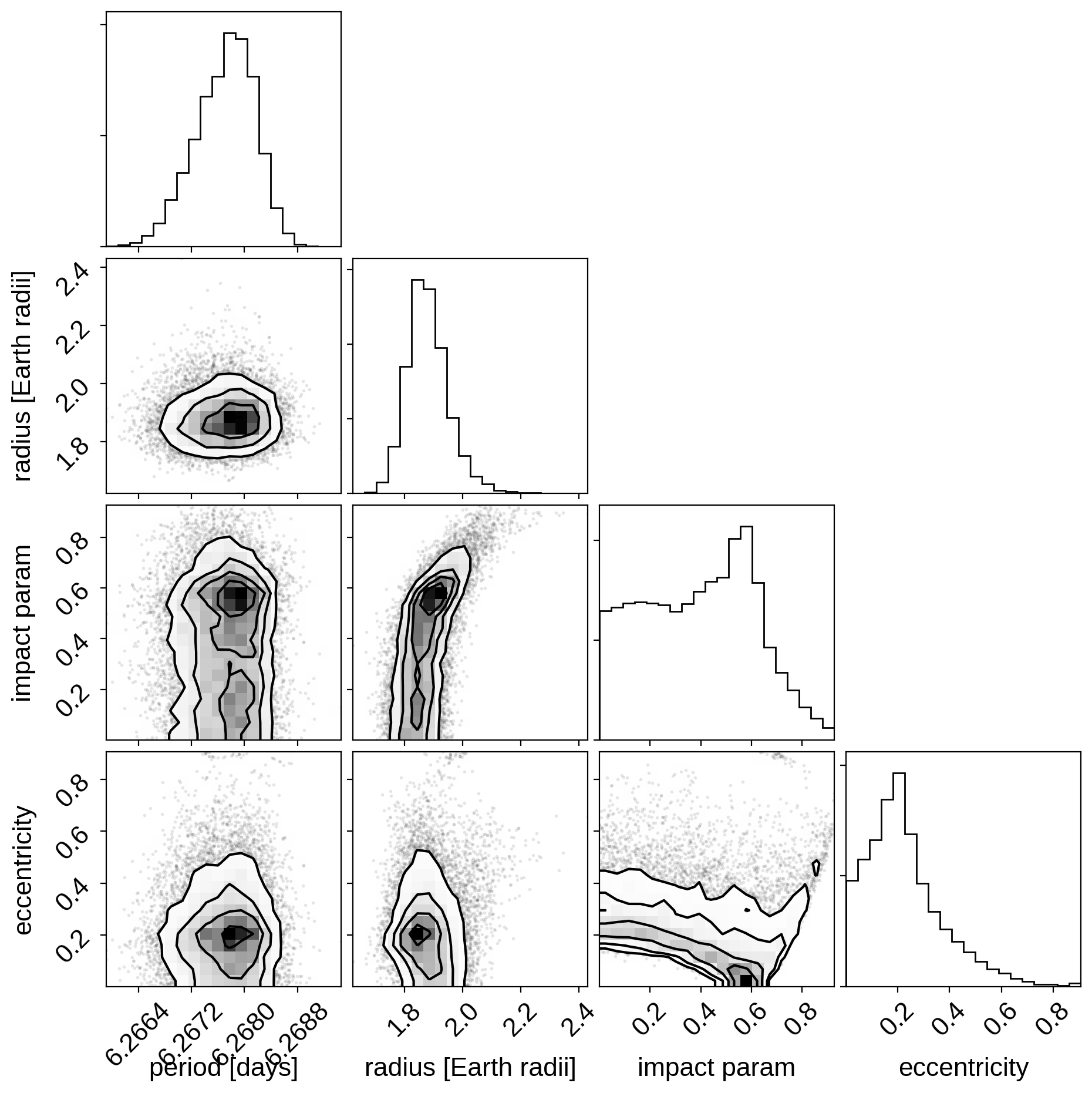Note

This tutorial was generated from an IPython notebook that can be downloaded here.

# Fitting TESS data¶

In this tutorial, we will reproduce the fits to the transiting planet in the Pi Mensae system discovered by Huang et al. (2018). The data processing and model are similar to the Putting it all together tutorial, but with a few extra bits like aperture selection and de-trending.

import numpy as np
import lightkurve as lk
import matplotlib.pyplot as plt

with tpf_file.hdu as hdu:
tpf = hdu.data

texp = tpf_hdr["FRAMETIM"] * tpf_hdr["NUM_FRM"]
texp /= 60.0 * 60.0 * 24.0
time = tpf["TIME"]
flux = tpf["FLUX"]
m = np.any(np.isfinite(flux), axis=(1, 2)) & (tpf["QUALITY"] == 0)
ref_time = 0.5 * (np.min(time[m]) + np.max(time[m]))
time = np.ascontiguousarray(time[m] - ref_time, dtype=np.float64)
flux = np.ascontiguousarray(flux[m], dtype=np.float64)

mean_img = np.median(flux, axis=0)
plt.imshow(mean_img.T, cmap="gray_r")
plt.title("TESS image of Pi Men")
plt.xticks([])
plt.yticks([]);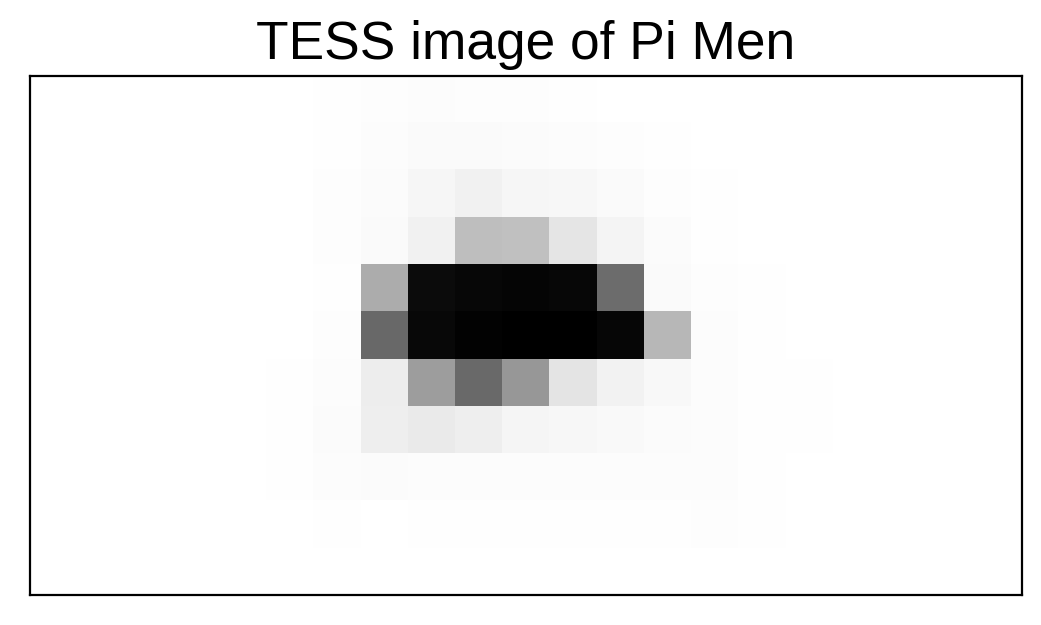## Aperture selection¶

Next, we’ll select an aperture using a hacky method that tries to minimizes the windowed scatter in the lightcurve (something like the CDPP).

from scipy.signal import savgol_filter

# Sort the pixels by median brightness
order = np.argsort(mean_img.flatten())[::-1]

# A function to estimate the windowed scatter in a lightcurve
smooth = savgol_filter(f, 1001, polyorder=5)
return 1e6 * np.sqrt(np.median((f / smooth - 1) ** 2))

# Loop over pixels ordered by brightness and add them one-by-one
# to the aperture
for i in range(10, 100):
msk = np.zeros_like(mean_img, dtype=bool)
msk[np.unravel_index(order[:i], mean_img.shape)] = True
scatters.append(scatter)

# Choose the aperture that minimizes the scatter

# Plot the selected aperture
plt.imshow(mean_img.T, cmap="gray_r")
plt.title("selected aperture")
plt.xticks([])
plt.yticks([]);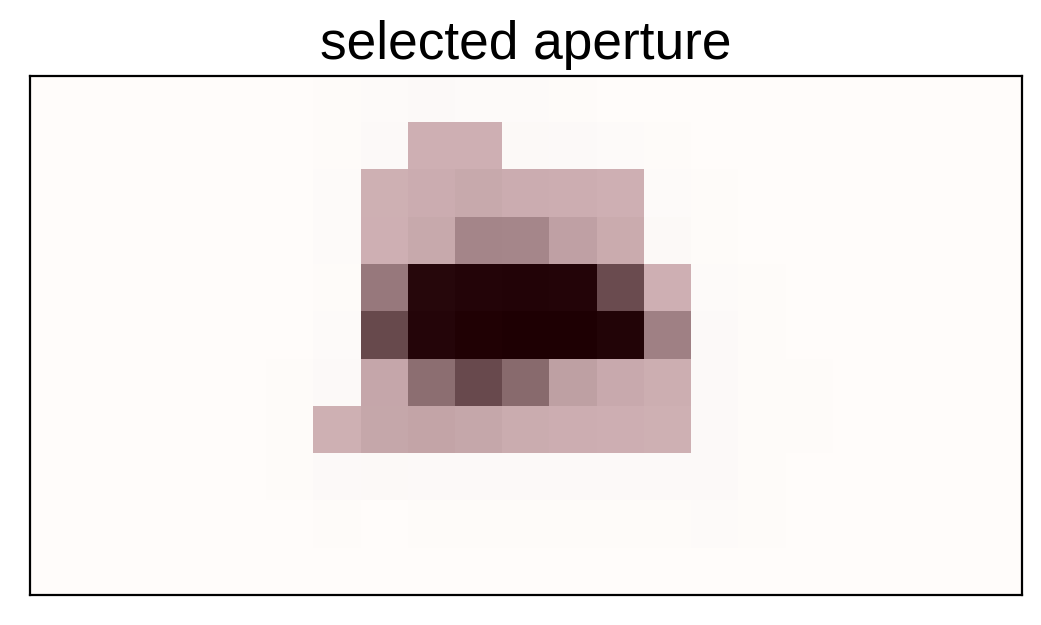This aperture produces the following light curve:

plt.figure(figsize=(10, 5))
sap_flux = (sap_flux / np.median(sap_flux) - 1) * 1e3
plt.plot(time, sap_flux, "k")
plt.xlabel("time [days]")
plt.ylabel("relative flux [ppt]")
plt.title("raw light curve")
plt.xlim(time.min(), time.max());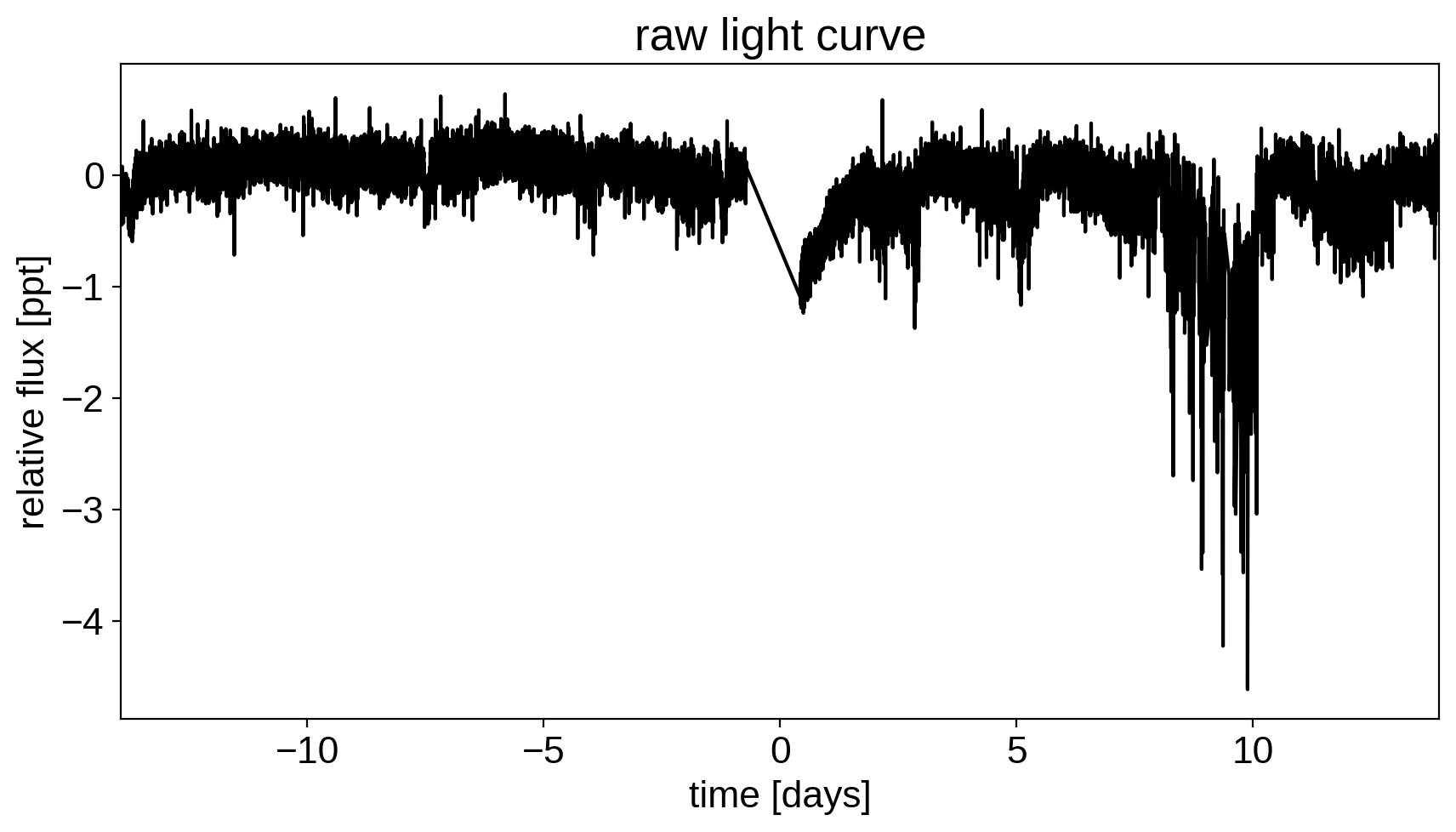## The transit model in PyMC3¶

The transit model, initialization, and sampling are all nearly the same as the one in Putting it all together.

import exoplanet as xo
import pymc3 as pm
import theano.tensor as tt

with pm.Model() as model:

# Parameters for the stellar properties
mean = pm.Normal("mean", mu=0.0, sd=10.0)

# Stellar parameters from Huang et al (2018)
M_star_huang = 1.094, 0.039
R_star_huang = 1.10, 0.023
BoundedNormal = pm.Bound(pm.Normal, lower=0, upper=3)
m_star = BoundedNormal("m_star", mu=M_star_huang, sd=M_star_huang)
r_star = BoundedNormal("r_star", mu=R_star_huang, sd=R_star_huang)

# Orbital parameters for the planets
logP = pm.Normal("logP", mu=np.log(bls_period), sd=1)
t0 = pm.Normal("t0", mu=bls_t0, sd=1)
logr = pm.Normal(
"logr",
sd=1.0,
mu=0.5 * np.log(1e-3 * np.array(bls_depth)) + np.log(R_star_huang),
)
r_pl = pm.Deterministic("r_pl", tt.exp(logr))
ror = pm.Deterministic("ror", r_pl / r_star)
b = xo.distributions.ImpactParameter("b", ror=ror)

# This is the eccentricity prior from Kipping (2013):
# https://arxiv.org/abs/1306.4982
ecc = xo.distributions.eccentricity.kipping13("ecc", testval=0.1)
omega = xo.distributions.Angle("omega")

# Transit jitter & GP parameters
logw0 = pm.Normal("logw0", mu=0, sd=10)

# Tracking planet parameters
period = pm.Deterministic("period", tt.exp(logP))

# Orbit model
orbit = xo.orbits.KeplerianOrbit(
r_star=r_star,
m_star=m_star,
period=period,
t0=t0,
b=b,
ecc=ecc,
omega=omega,
)

# Compute the model light curve using starry
light_curves = (
xo.LimbDarkLightCurve(u_star).get_light_curve(
)
* 1e3
)
light_curve = pm.math.sum(light_curves, axis=-1) + mean
pm.Deterministic("light_curves", light_curves)

# GP model for the light curve
kernel = xo.gp.terms.SHOTerm(log_Sw4=logSw4, log_w0=logw0, Q=1 / np.sqrt(2))
pm.Deterministic("gp_pred", gp.predict())

# Fit for the maximum a posteriori parameters, I've found that I can get
# a better solution by trying different combinations of parameters in turn
if start is None:
start = model.test_point
map_soln = xo.optimize(start=start, vars=[logs2, logSw4, logw0])
map_soln = xo.optimize(start=map_soln, vars=[logr])
map_soln = xo.optimize(start=map_soln, vars=[b])
map_soln = xo.optimize(start=map_soln, vars=[logP, t0])
map_soln = xo.optimize(start=map_soln, vars=[u_star])
map_soln = xo.optimize(start=map_soln, vars=[logr])
map_soln = xo.optimize(start=map_soln, vars=[b])
map_soln = xo.optimize(start=map_soln, vars=[ecc, omega])
map_soln = xo.optimize(start=map_soln, vars=[mean])
map_soln = xo.optimize(start=map_soln, vars=[logs2, logSw4, logw0])
map_soln = xo.optimize(start=map_soln)

return model, map_soln

model0, map_soln0 = build_model()

optimizing logp for variables: [logw0, logSw4, logs2]
20it [00:09,  2.20it/s, logp=1.264290e+04]
message: Optimization terminated successfully.
logp: 12406.06185575557 -> 12642.89955925584
optimizing logp for variables: [logr]
207it [00:03, 66.50it/s, logp=1.268195e+04]
message: Desired error not necessarily achieved due to precision loss.
logp: 12642.899559255851 -> 12681.951246651677
optimizing logp for variables: [b, logr, r_star]
64it [00:01, 33.35it/s, logp=1.294934e+04]
message: Desired error not necessarily achieved due to precision loss.
logp: 12681.951246651677 -> 12949.339148287803
optimizing logp for variables: [t0, logP]
99it [00:02, 48.84it/s, logp=1.296374e+04]
message: Desired error not necessarily achieved due to precision loss.
logp: 12949.339148287796 -> 12963.740755133214
optimizing logp for variables: [u_star]
12it [00:01, 11.31it/s, logp=1.296662e+04]
message: Optimization terminated successfully.
logp: 12963.740755133207 -> 12966.624941258444
optimizing logp for variables: [logr]
9it [00:01,  6.81it/s, logp=1.296682e+04]
message: Optimization terminated successfully.
logp: 12966.624941258455 -> 12966.818870393383
optimizing logp for variables: [b, logr, r_star]
16it [00:01, 11.19it/s, logp=1.296694e+04]
message: Optimization terminated successfully.
logp: 12966.818870393383 -> 12966.944430577478
optimizing logp for variables: [omega, ecc]
23it [00:01, 15.09it/s, logp=1.298782e+04]
message: Optimization terminated successfully.
logp: 12966.944430577478 -> 12987.822019225678
optimizing logp for variables: [mean]
5it [00:01,  3.06it/s, logp=1.298785e+04]
message: Optimization terminated successfully.
logp: 12987.822019225663 -> 12987.85030612374
optimizing logp for variables: [logw0, logSw4, logs2]
47it [00:01, 27.03it/s, logp=1.299865e+04]
message: Desired error not necessarily achieved due to precision loss.
logp: 12987.85030612374 -> 12998.653500794684
optimizing logp for variables: [logSw4, logw0, logs2, omega, ecc, ecc_beta, ecc_alpha, b, logr, t0, logP, r_star, m_star, u_star, mean]
111it [00:01, 82.65it/s, logp=1.305483e+04]
message: Desired error not necessarily achieved due to precision loss.
logp: 12998.653500794699 -> 13054.830649513216


Here’s how we plot the initial light curve model:

def plot_light_curve(soln, mask=None):

fig, axes = plt.subplots(3, 1, figsize=(10, 7), sharex=True)

ax = axes
gp_mod = soln["gp_pred"] + soln["mean"]
ax.legend(fontsize=10)
ax.set_ylabel("relative flux [ppt]")

ax = axes
for i, l in enumerate("b"):
mod = soln["light_curves"][:, i]
ax.legend(fontsize=10, loc=3)
ax.set_ylabel("de-trended flux [ppt]")

ax = axes
mod = gp_mod + np.sum(soln["light_curves"], axis=-1)
ax.axhline(0, color="#aaaaaa", lw=1)
ax.set_ylabel("residuals [ppt]")
ax.set_xlabel("time [days]")

return fig

plot_light_curve(map_soln0);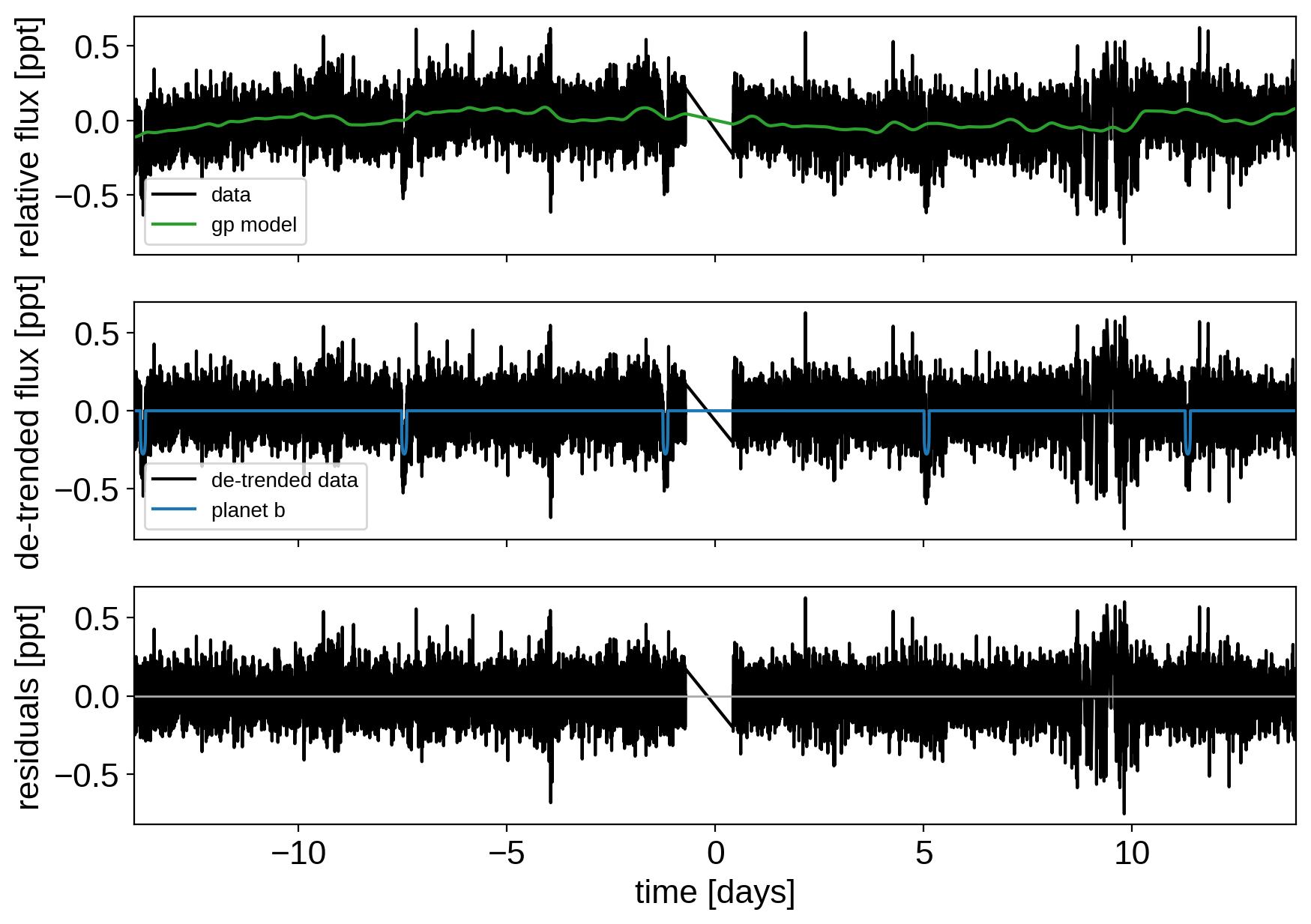As in the Putting it all together tutorial, we can do some sigma clipping to remove significant outliers.

mod = (
map_soln0["gp_pred"]
+ map_soln0["mean"]
+ np.sum(map_soln0["light_curves"], axis=-1)
)
resid = y - mod
rms = np.sqrt(np.median(resid ** 2))
mask = np.abs(resid) < 5 * rms

plt.figure(figsize=(10, 5))
plt.plot(x, resid, "k", label="data")
plt.axhline(0, color="#aaaaaa", lw=1)
plt.ylabel("residuals [ppt]")
plt.xlabel("time [days]")
plt.legend(fontsize=12, loc=3)
plt.xlim(x.min(), x.max());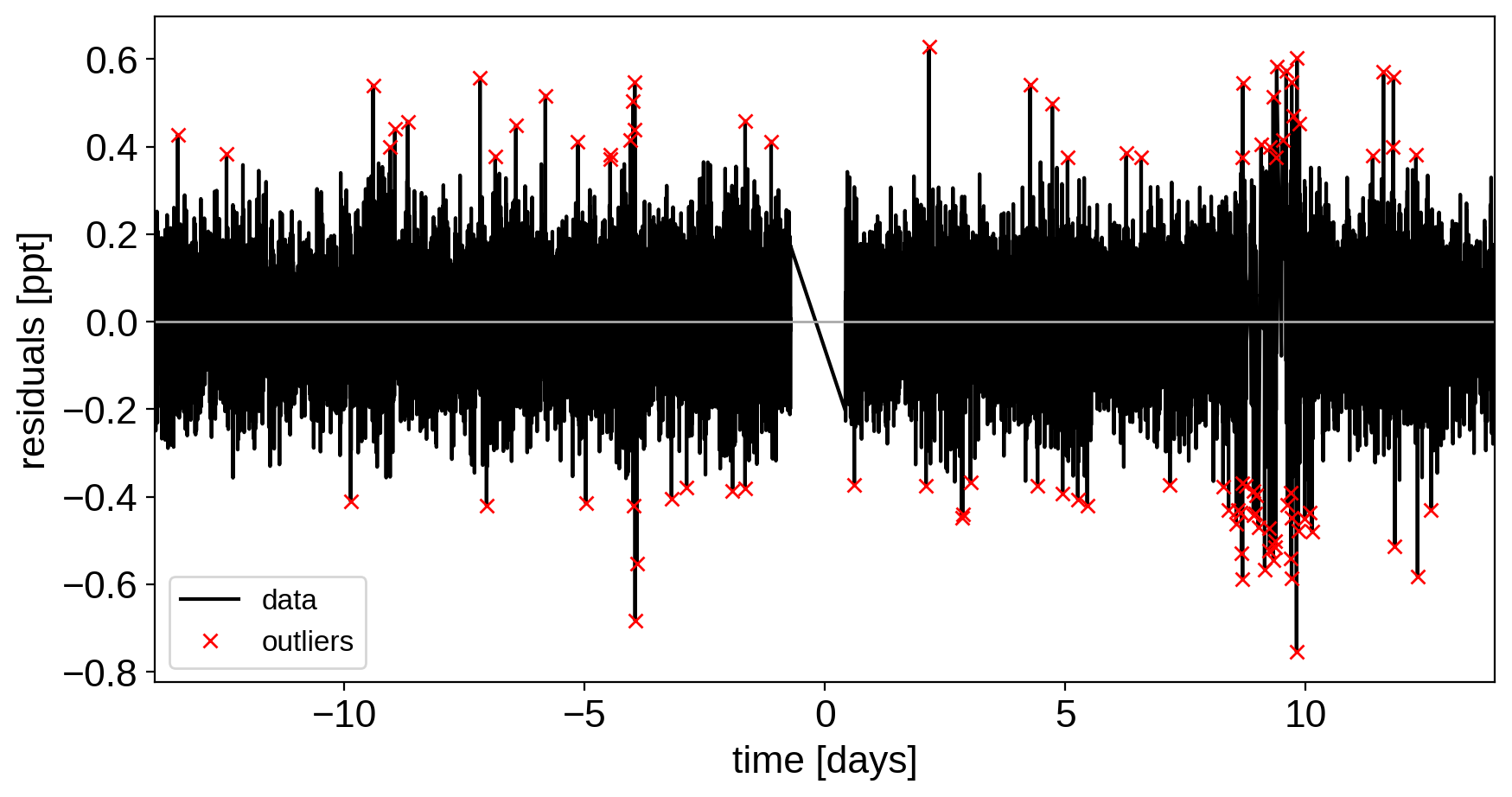And then we re-build the model using the data without outliers.

model, map_soln = build_model(mask, map_soln0)

optimizing logp for variables: [logw0, logSw4, logs2]
15it [00:01,  9.27it/s, logp=1.374030e+04]
message: Optimization terminated successfully.
logp: 13709.337756357809 -> 13740.297129156785
optimizing logp for variables: [logr]
8it [00:01,  5.54it/s, logp=1.374032e+04]
message: Optimization terminated successfully.
logp: 13740.297129156788 -> 13740.31722986988
optimizing logp for variables: [b, logr, r_star]
14it [00:01,  8.96it/s, logp=1.374032e+04]
message: Optimization terminated successfully.
logp: 13740.31722986988 -> 13740.31831713007
optimizing logp for variables: [t0, logP]
146it [00:02, 57.04it/s, logp=1.374033e+04]
message: Desired error not necessarily achieved due to precision loss.
logp: 13740.318317130062 -> 13740.326927416274
optimizing logp for variables: [u_star]
8it [00:01,  4.93it/s, logp=1.374035e+04]
message: Optimization terminated successfully.
logp: 13740.326927416267 -> 13740.350391911023
optimizing logp for variables: [logr]
8it [00:01,  4.53it/s, logp=1.374035e+04]
message: Optimization terminated successfully.
logp: 13740.35039191103 -> 13740.353904884088
optimizing logp for variables: [b, logr, r_star]
85it [00:00, 113.98it/s, logp=1.374036e+04]
message: Desired error not necessarily achieved due to precision loss.
logp: 13740.353904884088 -> 13740.362784306773
optimizing logp for variables: [omega, ecc]
125it [00:01, 98.94it/s, logp=1.374036e+04]
message: Desired error not necessarily achieved due to precision loss.
logp: 13740.362784306773 -> 13740.362835323785
optimizing logp for variables: [mean]
5it [00:01,  2.93it/s, logp=1.374037e+04]
message: Optimization terminated successfully.
logp: 13740.362835323785 -> 13740.366005030273
optimizing logp for variables: [logw0, logSw4, logs2]
9it [00:01,  6.95it/s, logp=1.374037e+04]
message: Optimization terminated successfully.
logp: 13740.366005030273 -> 13740.36600761282
optimizing logp for variables: [logSw4, logw0, logs2, omega, ecc, ecc_beta, ecc_alpha, b, logr, t0, logP, r_star, m_star, u_star, mean]
91it [00:02, 34.39it/s, logp=1.374038e+04]
message: Desired error not necessarily achieved due to precision loss.
logp: 13740.366007612805 -> 13740.377939831247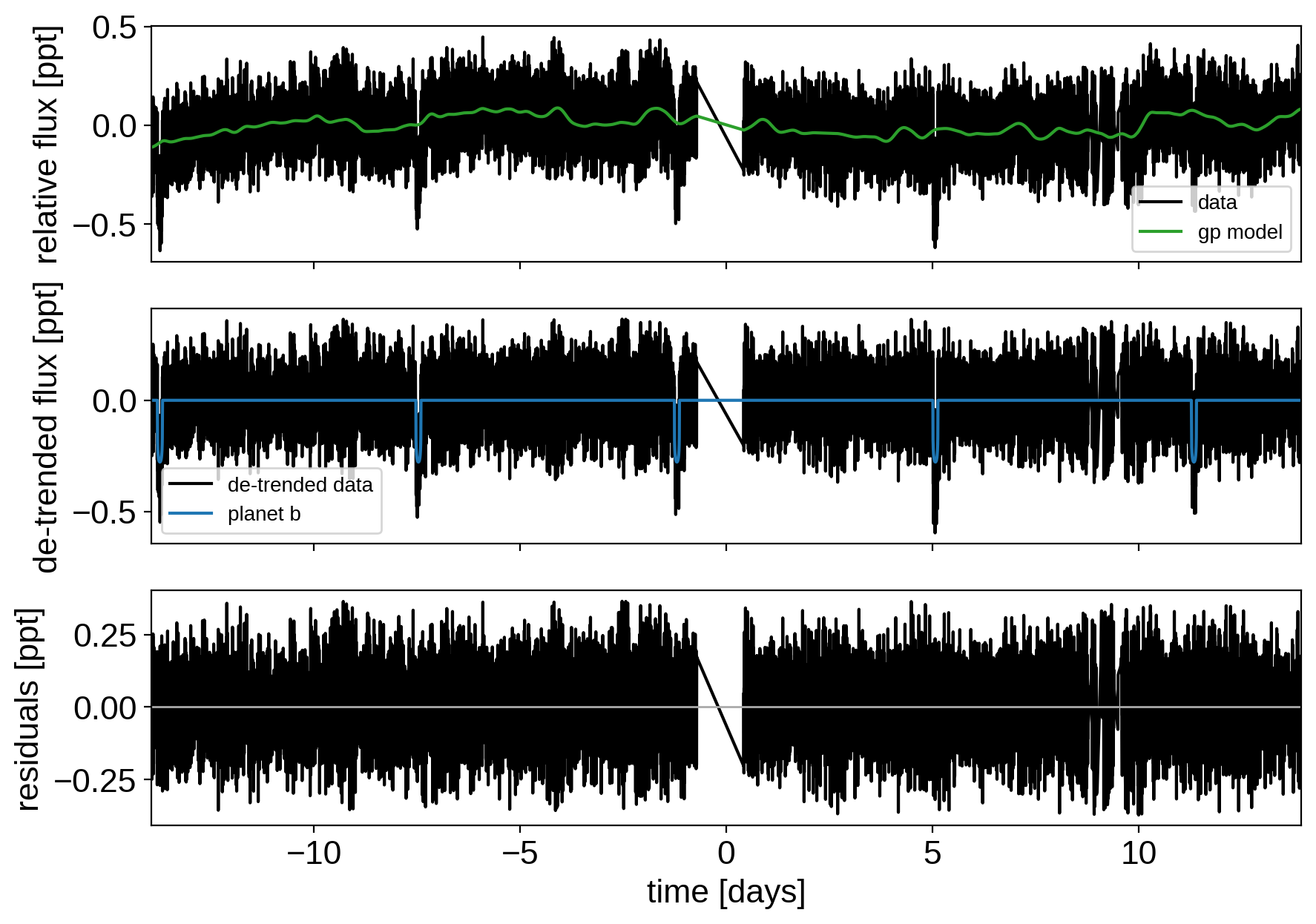Now that we have the model, we can sample:

np.random.seed(42)
with model:
trace = pm.sample(
tune=5000,
draws=3000,
start=map_soln,
chains=4,
step=xo.get_dense_nuts_step(target_accept=0.9),
)

Multiprocess sampling (4 chains in 4 jobs)
NUTS: [logSw4, logw0, logs2, omega, ecc, ecc_beta, ecc_alpha, b, logr, t0, logP, r_star, m_star, u_star, mean]
Sampling 4 chains: 100%|██████████| 32000/32000 [2:42:04<00:00,  1.01draws/s]
The number of effective samples is smaller than 25% for some parameters.

pm.summary(
trace,
varnames=[
"logw0",
"logSw4",
"logs2",
"omega",
"ecc",
"r_pl",
"b",
"t0",
"logP",
"r_star",
"m_star",
"u_star",
"mean",
],
)

mean sd mc_error hpd_2.5 hpd_97.5 n_eff Rhat
logw0 1.176557 0.135296 1.451517e-03 0.914931 1.443757 8688.248464 0.999916
logSw4 -2.105827 0.317614 3.047235e-03 -2.701237 -1.464207 10667.609961 0.999894
logs2 -4.382870 0.010754 1.055636e-04 -4.404120 -4.362122 11519.863118 0.999949
omega 0.648294 1.715356 3.512658e-02 -2.809296 3.141469 2329.886518 1.000512
ecc 0.222420 0.143264 3.047271e-03 0.000121 0.509765 2381.265132 1.001254
r_pl 0.017243 0.000648 1.334355e-05 0.015987 0.018522 2047.263980 1.001190
b 0.390768 0.221809 5.168899e-03 0.000139 0.736551 1494.738718 1.001950
t0 -1.197385 0.000680 1.596423e-05 -1.198662 -1.196055 1929.200269 1.001265
logP 1.835411 0.000069 7.772674e-07 1.835271 1.835534 6657.291370 1.000026
r_star 1.098913 0.022882 2.436166e-04 1.055207 1.144789 10624.471452 0.999884
m_star 1.095748 0.039422 3.385010e-04 1.017889 1.170311 11559.600340 1.000214
u_star__0 0.204246 0.169537 2.327905e-03 0.000086 0.542679 6008.558020 1.000173
u_star__1 0.447711 0.275453 4.260404e-03 -0.100902 0.936185 5088.343252 1.000099
mean -0.001239 0.008905 9.158234e-05 -0.019270 0.015879 9237.028735 0.999898

## Results¶

After sampling, we can make the usual plots. First, let’s look at the folded light curve plot:

# Compute the GP prediction
gp_mod = np.median(trace["gp_pred"] + trace["mean"][:, None], axis=0)

# Get the posterior median orbital parameters
p = np.median(trace["period"])
t0 = np.median(trace["t0"])

# Plot the folded data
x_fold = (x[mask] - t0 + 0.5 * p) % p - 0.5 * p
plt.plot(x_fold, y[mask] - gp_mod, ".k", label="data", zorder=-1000)

# Overplot the phase binned light curve
bins = np.linspace(-0.41, 0.41, 50)
denom, _ = np.histogram(x_fold, bins)
num, _ = np.histogram(x_fold, bins, weights=y[mask])
denom[num == 0] = 1.0
plt.plot(0.5 * (bins[1:] + bins[:-1]), num / denom, "o", color="C2", label="binned")

# Plot the folded model
inds = np.argsort(x_fold)
inds = inds[np.abs(x_fold)[inds] < 0.3]
pred = trace["light_curves"][:, inds, 0]
pred = np.percentile(pred, [16, 50, 84], axis=0)
plt.plot(x_fold[inds], pred, color="C1", label="model")
art = plt.fill_between(
x_fold[inds], pred, pred, color="C1", alpha=0.5, zorder=1000
)
art.set_edgecolor("none")

# Annotate the plot with the planet's period
txt = "period = {0:.5f} +/- {1:.5f} d".format(
np.mean(trace["period"]), np.std(trace["period"])
)
plt.annotate(
txt,
(0, 0),
xycoords="axes fraction",
xytext=(5, 5),
textcoords="offset points",
ha="left",
va="bottom",
fontsize=12,
)

plt.legend(fontsize=10, loc=4)
plt.xlim(-0.5 * p, 0.5 * p)
plt.xlabel("time since transit [days]")
plt.ylabel("de-trended flux")
plt.xlim(-0.15, 0.15);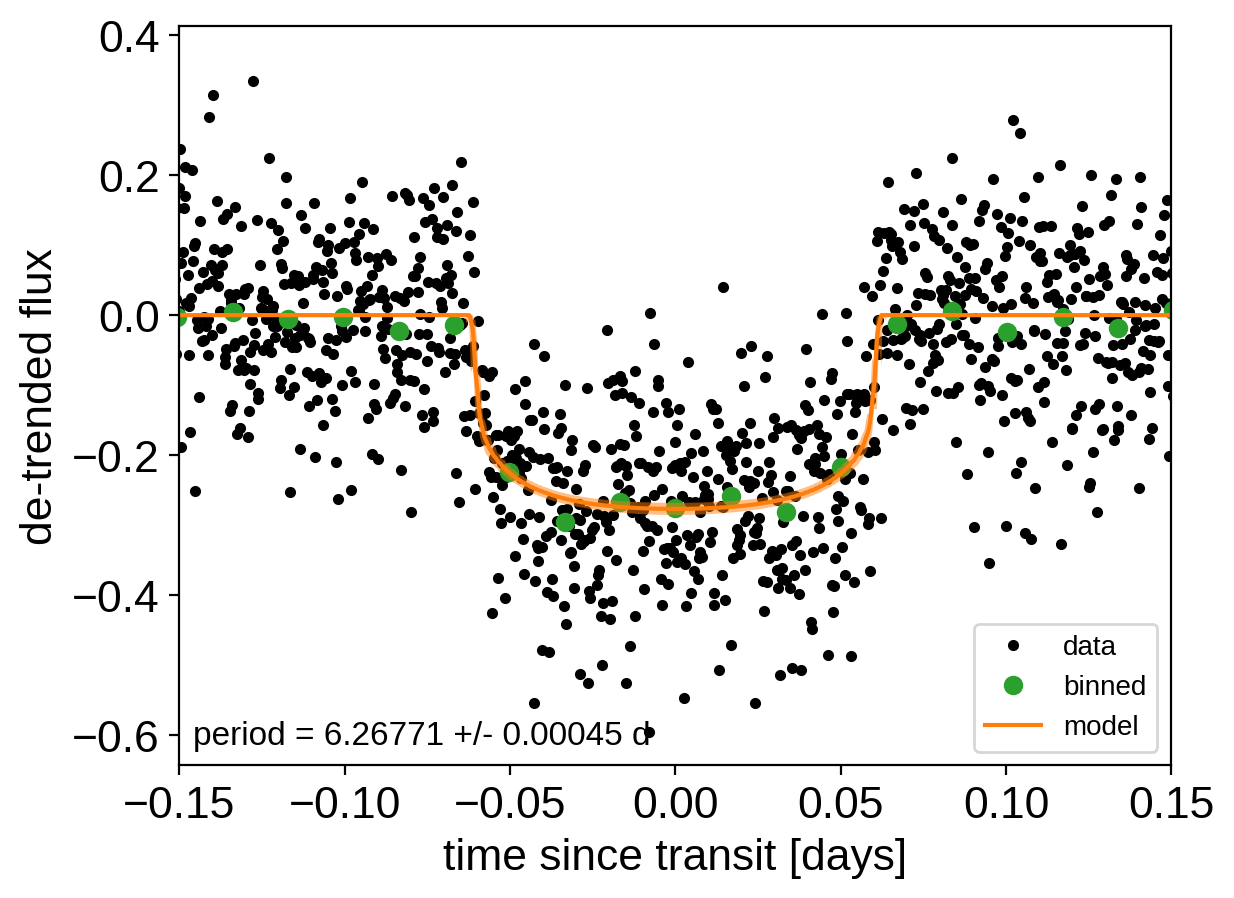And a corner plot of some of the key parameters:

import corner
import astropy.units as u

varnames = ["period", "b", "ecc", "r_pl"]
samples = pm.trace_to_dataframe(trace, varnames=varnames)